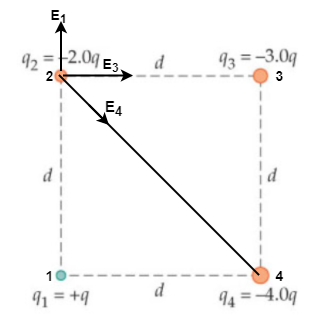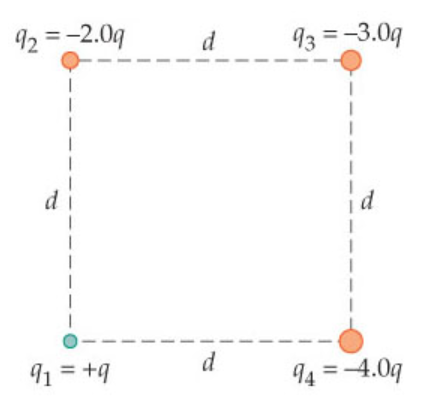# Problem: Find the direction and magnitude of the net electrostatic force exerted on the point charge q2 in the figure. Let q=+2.6mu and d=35cm. Answer in Newtons. Please show all your work.

###### FREE Expert Solution

The electric force is given by:

$\overline{){{\mathbf{F}}}_{{\mathbf{e}}}{\mathbf{=}}{\mathbf{q}}{\mathbf{E}}}$, where q is the charge and E is the electric field.

Electric field:

$\overline{){\mathbf{E}}{\mathbf{=}}\frac{\mathbf{k}\mathbf{q}}{{\mathbf{r}}^{\mathbf{2}}}}$, where k is Coulomb's constant and r is the distance from the point to the charge q.

We can visualize the electric fields in the diagram as follows:Now,

91% (110 ratings)###### Problem Details

Find the direction and magnitude of the net electrostatic force exerted on the point charge q2 in the figure. Let q=+2.6mu and d=35cm. Answer in Newtons. Please show all your work.